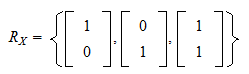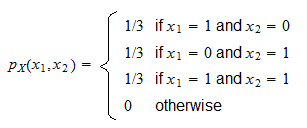StatLect

Joint probability mass function

The joint probability mass function is a function that completely characterizes the distribution of a discrete random vector. When evaluated at a given point, it gives the probability that the realization of the random vector will be equal to that point.Synonyms and acronyms

The term joint probability function is often used as a synonym. Sometimes, the abbreviation joint pmf is used.

Definition

The following is a formal definition.

Definition Letbe adiscrete random vector. Its joint probability mass function is a functionsuch thatwhereis the probability that the random vectortakes the value.

Example

Supposeis adiscrete random vector and that its support (the set of values it can take) is:If the three values have the same probability, then the joint probability mass function is:Denoting the two components ofbyand, its joint pmf can also be written using the following alternative notation:More details

This is a glossary entry. For a thorough discussion of joint pmfs, go to the lecture entitled Random vectors, where discrete random vectors are introduced and you can also find some exercises involving joint pmfs.